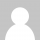# 如何避免产生赋值语句

## 用正确的方式初始化

``````// 错误       |  // 正确
int x;        |
// ...        |  // ...
x = init();   |  int x = init();
``````

“正确”方式的主要优点是你能很方便的浏览`x`的定义的同时知道它的值。这样也能保证`x`始终处在一个固定变量状态，大多数的编译器都能检测到这种状态。其次，这样可以使代码减少冗余。

“错误”方式之所以存在完全是因为很多老式的编程语言都强制要求在程序的开始处先声明变量。这样编译器好处理。但现在这已经不是问题了，即使在C语言里。

## 构造新数据

``````// 错误          |  // 正确
int x = init();  |  int x = init();
// ...           |  // ...
x = something(); |  int y = something();
``````

## 用函数，不要用过程

``````// 错误                  |  // 正确
void to_utf8(string s);  |  string to_utf8(string s);
|
// ...                   |  // ...
|
string s1 = latin();     |  use_string(to_utf8(latin()))
to_utf8(s1);             |
use_string(s1);          |
``````

“正确”方式使用的是一个普通的数字函数：它接受输入值，返回计算后的值。另一边，“错误”方式使用了过程。跟函数不一样，过程不仅会影响返回的结果，还能影响其它数据，例如，过程中可以修改它的参数值。这会使这些参数很容易被污染。所以，当能够使用函数的时候，尽量不要使用过程。你的程序这样会变得更简单。这种技巧可以让你避免去思考如何去做(变换一个字符串)，而是如何被做(一个变换了的字符串)。要着眼于最终结果，而不是处理过程。

“错误”方式之所以存在完全是由于许多老的编程语言很难处理复杂的返回值。你只能返回单个数字。所以，当需要一个内容更丰富的返回值时，你只能在过 程中达到这个目的。而真正的返回值通常是一些简单的错误标号代码。然而现在不同了，返回复杂的结果已经不再是个问题。即使是在C语言里你也可以返回复杂的 结果。

## 固化你的对象

``````// 非常非常错误
class Point
{
public:
// constructor
Point() { x = 0; y = 0; }

float get_x() { return x; }
float get_y() { return y; }

void set_x(float new_x) { x = new_x; }
void set_y(float new_y) { y = new_y; }

move(Point p) {
x = x + p.x;
y = y + p.y;
}
private:
float x; float y;
};
``````

• 构造函数直接把`x``y` 初始化成0了。如果你希望它是其它值，你还需要手工的设置。你不能初始时做到这些
• 操作一个点的缺省方法就是修改它。这是一种赋值操作。你被限制了创建一个新值
• `set_x()`, `set_y()`, 和 `move()` 方法现场修改这个对象。这些是过程, 不是函数
• `x` (和 `y`) 是私有的，但你可以通过`get_x()``set_x()`操作它们。所以，你认为你是封装了它们，而实际上没有。
• `move()`这个方法不需要放在`Point`类里。放在类里使类的体积变大，影响理解和使用。

``````// 正确的
class Point
{
public:
// constructor
Point (float x, float y) {
_x = x; _y = y;
}

x() { return x; }
y() { return y; }

private:
float _x; float _y
}

Point move(Point p1, Point p2) {
return Point(p1.x() + p2.x(),
p1.y() + p2.y());
}
``````

## 使用纯功能性数据结构

``````┌───┬───┐   ┌───┬───┐
│ x │  ───> │ y │  ───> empty
└───┴───┘   └───┴───┘
``````

``````-- Haskell
-- A list is either the Empty list,
-- or it contains an Int and a List
data List = Empty
| NotEmpty Int List

-- utility functions

is_empty Empty         = true
is_empty NotEmpty x xs = false

head NotEmpty x xs = x

tail Empty         = error
tail notEmpty x xs = xs
``````
``````// Java(ish)
class List
{
public:
// constructors
List() { _is_empty = true; }
List(int i, List next) {
_i        = i;
_next     = next;
_is_empty = false;
}

bool is_empty() { return _is_empty; }

if (_is_empty) error();
return _i;
}

List tail() {
if (_is_empty) error();
return _next;
}

private:
int  _i;
List _next;
bool _is_empty;
}
``````

``````    ┌───┬───┐   ┌───┬───┐
l = │ x │  ───> │ y │  ───> empty
└───┴───┘   └───┴───┘

i = 42
``````

``````     ┌───┬───┐
l2 = │ i │   │
└───┴─│─┘
│
│   ┌───┬───┐   ┌───┬───┐
l  =       └──>│ x │  ───> │ y │  ───> empty
└───┴───┘   └───┴───┘
``````

``````List l  = List(x, List(y, List()));
int  i  = 42;

List l2 = List(i, l); // cheap
``````

`l` 仍然存在，不可变，而新建的`l2`只是多了一个新建的单元。类似的，删除顶部的元素也是不费任何资源的容易。

## 当我们不能这样做时

``````array pure_sort (array a)
{
array a2 = copy(a);
quicksort(a2); // modify a2, nothing else
return a2;
}
``````

``````// 错误

Point p(0, 0);
wile(true) // loop forever
{
p = move(p, get_mouse_movement());

if (p.x() < 0   ) p = Point(0    , p.y());
if (p.x() > 1024) p = Point(1024 , p.y());
if (p.y() < 0   ) p = Point(p.x(), 0    );
if (p.y() > 768 ) p = Point(p.x(), 768  );

draw(p);
}
``````

``````// 正确

point smart_move(point p, point mouse_movement)
{
float x = p.x() < 0    ? 0
: p.x() > 1024 ? 1024
:                p.x();

float y = p.y() < 0   ? 0
: p.y() > 768 ? 768
:               p.y();

return Point(x, y);
}

// 主程序
Point p(0, 0);
wile(true) // loop forever
{
p = smart_move(p, get_mouse_movement());
draw(p);
}
``````

``````// 这样也不是很差

point smart_move(point p, point mouse_movement)
{
float x = p.x();
float y = p.y();

if (x < 0   ) x = 0;
if (x > 1024) x = 1024;
if (y < 0   ) y = 0;
if (y > 768 ) y = 768;

return Point(x, y);
}
``````

## 结论

### 5 Responses to 如何避免产生赋值语句

1.say says:

博主，标题《如果避免产生赋值语句 》是不是错了
应该是如何吧？

•花非花 says:

正是，谢谢提醒

2.rotar says:

好象有些把面向对象编程向函数式编程方面带的意思，代码部分有些地方排版乱，是我的浏览器的原因么

3.tombai says:

bocai.zgfeng.cn

4.tombai says:

bbs.zgfeng.cn
baidu.zgfeng.cn
rc.zgfeng.cn
baike.zgfeng.cn
weixin.zgfeng.cn
bocai.zgfeng.cn
ailehui.com.cn
688td.com
jinse1hao.com
seo.seoseo.org.cn
seoseo.net.cn
zgh.mobi